Ezigrade (version 2)

# Smooth Surface - Multiple Sections

This scenario is very similar to the previous tutorial were we had 2 planes and a smooth solution joined together. Normally a "Variable" solution allows you to create a solution that would use less sections as the constraints can adapt to the job. In this example we have two distinct water flows to achieve and for this we need two seperate sections.

This example uses the data "grading2.cdsdat". Look in the "My Documents/Ezigrade" folder. This example is already setup with two sections as below. You will notice that we have rotated the section axes so that the Y direction lies in the distance that we want water to flow. You can also see that there is an existing drain towards the left edge of Section1 that we no longer require.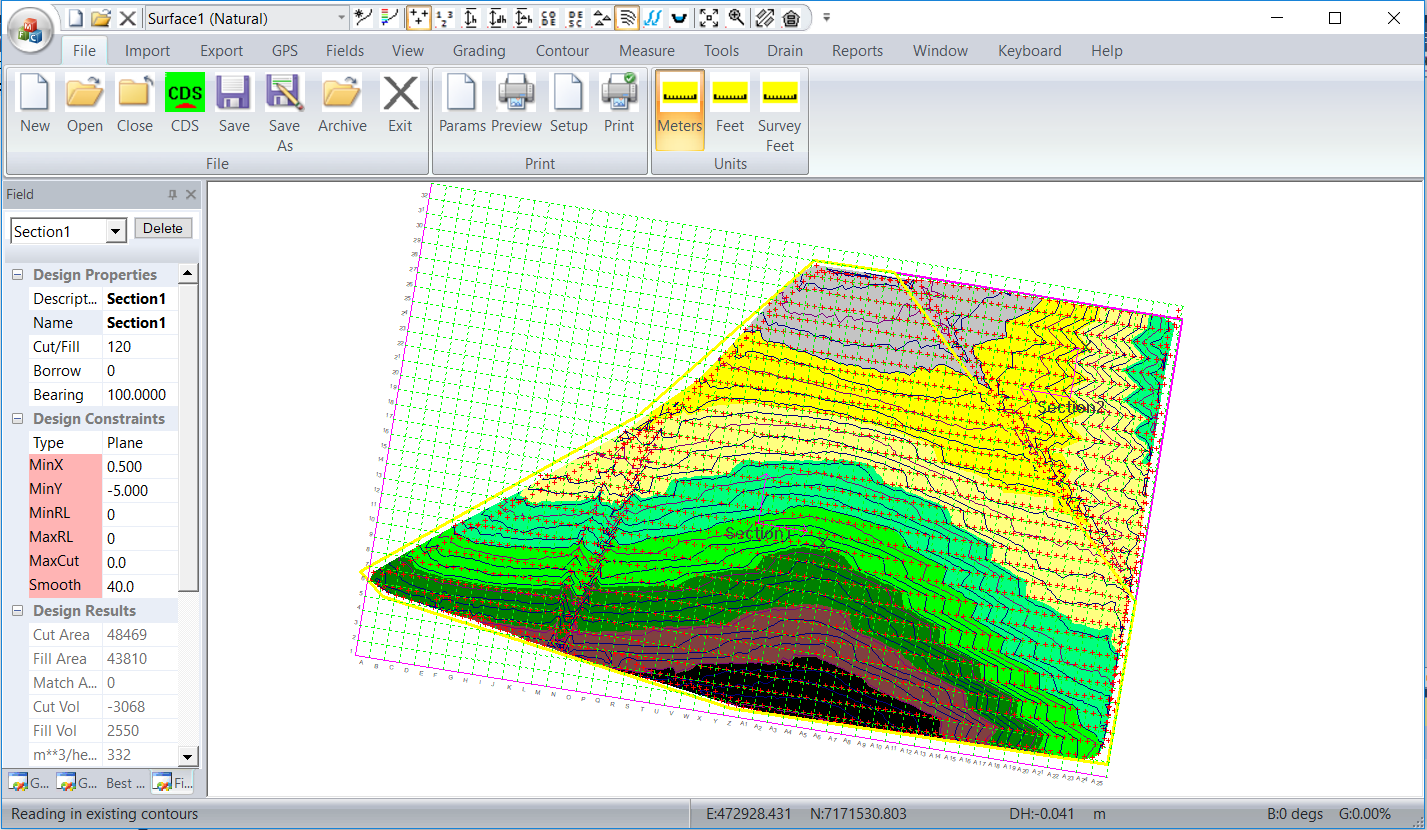We have set the job up as follows. Section 1 we will constrain the Y grades to lie between -.5 and -5%. The grade is negative as this means the water is flowing downhill. If you dont like negative numbers you can spin the axis around 180 degrees so we are looking uphill. Section 2 we are setting the Y grade between -1 and -4%. Normally the best way to approach the situation is to put in the minimum number of constarints and add constraints as we go; to refine the solution.

For a first solution we have clicked on the Grading -> Grid Based and used a grid size of 10m. We have the following and for reference the volumes for section1 are -1724/1437 for cut and fill respectively. You will notice that the existing drain is still visible and that particularly in section2 that the design is wavy.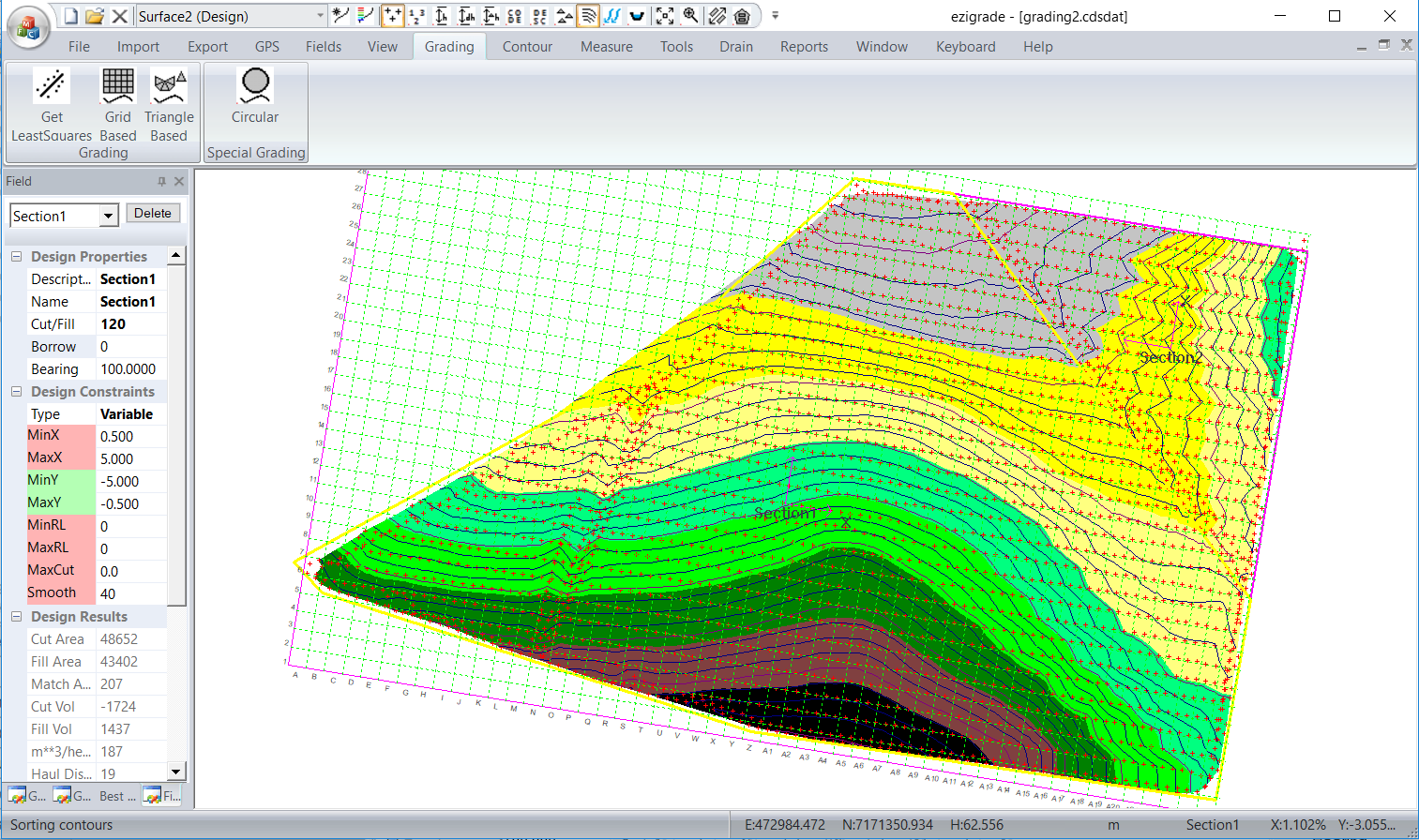Lets compare what we get with the triangle based solution. Running Grading -> Triangle Based gives us the following. You will notice that the result is generally more wavy and there are a couple of issues around the section line between section1 and section2. The cut fill volumes are -691/575 respectively which is significantly lower than the grid based solution. The grid based only picks up data that falls on the grid points so we tend to get a smoothing effect. The triangle based solution uses every triangle in the job so is more rigorous. In particular you will notice that the existing drain outline is still clearly visible. The grid based soltion has one advantage in that processing time is normally less as we are only using a subset of points.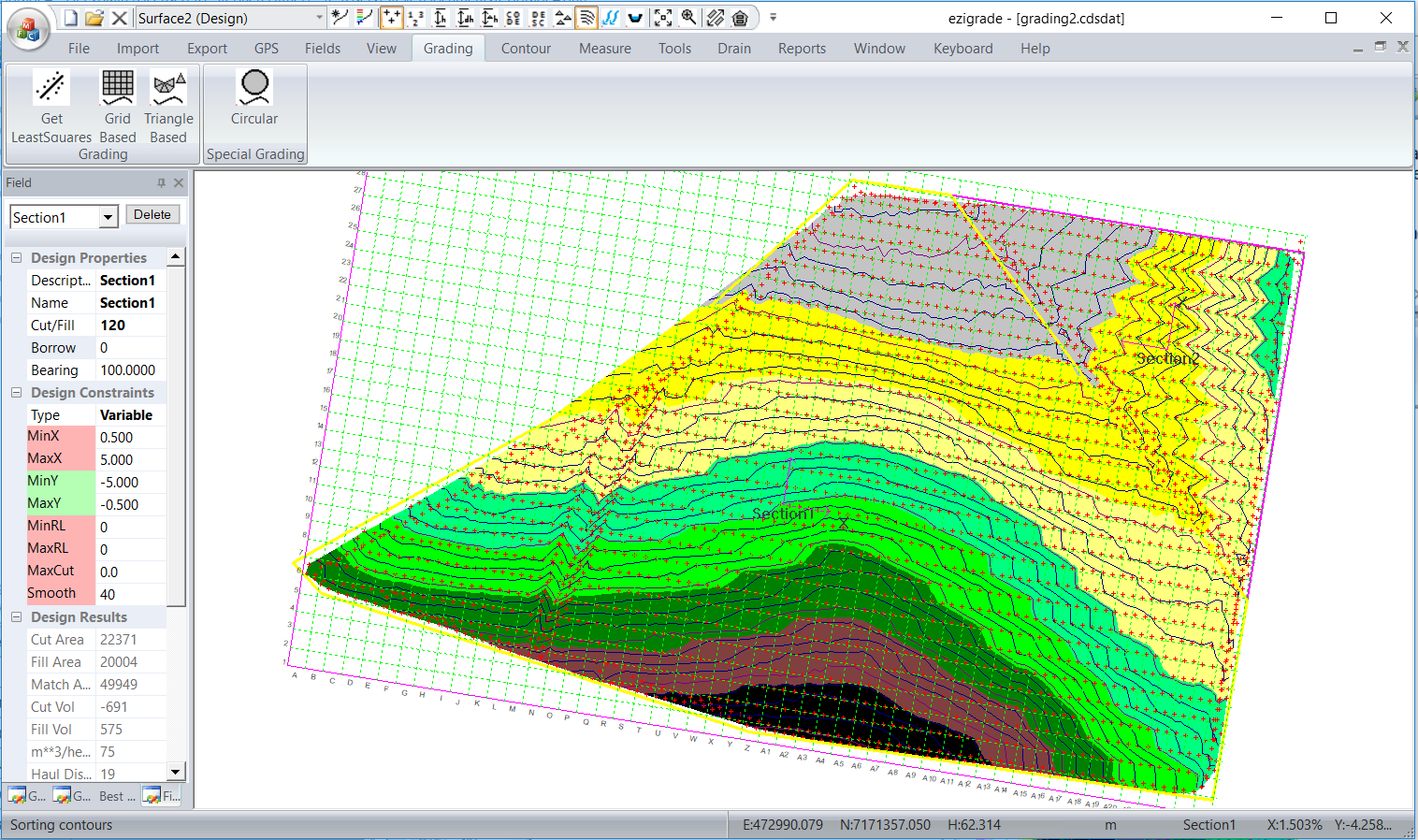Looking at the triangle based solution. We could clean up the existing drain a bit by constraining X grades. Similarly for Section2 - by restaraing X grades we may be able to alleviate the waviness. Running again after adding constraints in X directions.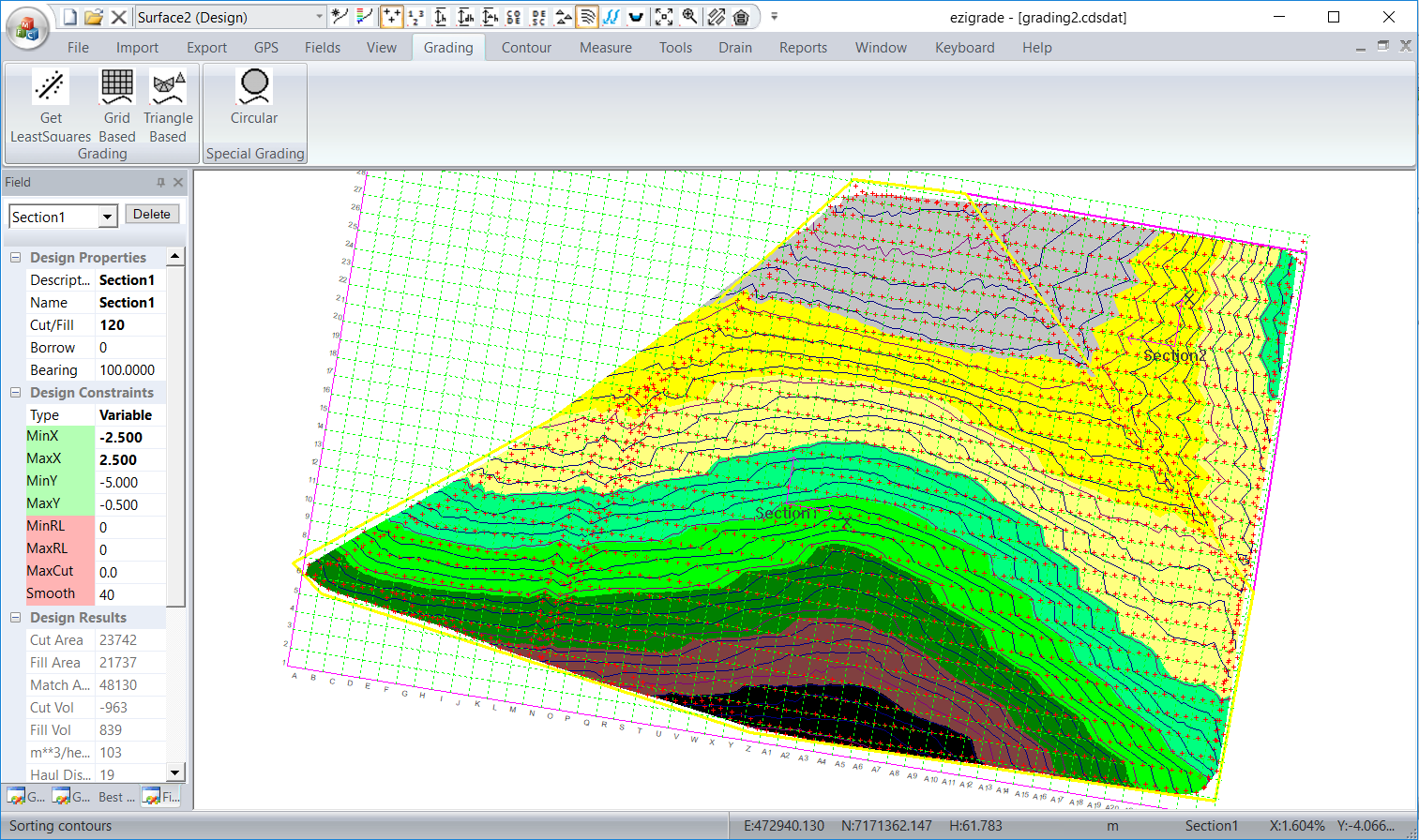We suggest that you experiment with these constraints until you are close. The final step is to include some smoothing into the equation. Make sure smooth option is clicked green and we have tried a distance of 40m on both sections. However there is nothing wrong with varying the smoothing value between sections. In this example you may choose a larger value for section2 as it is more "wavy" than section1. Running again we get:The volumes have certainly increased so that we could probably reduce the smoothing distance. However the existing drain is still visible and is affecting the final design. I would create a couple more sections so that the existing drain is in it's own section. I would then constrain the X values here to "fix" the drain.

Something like below: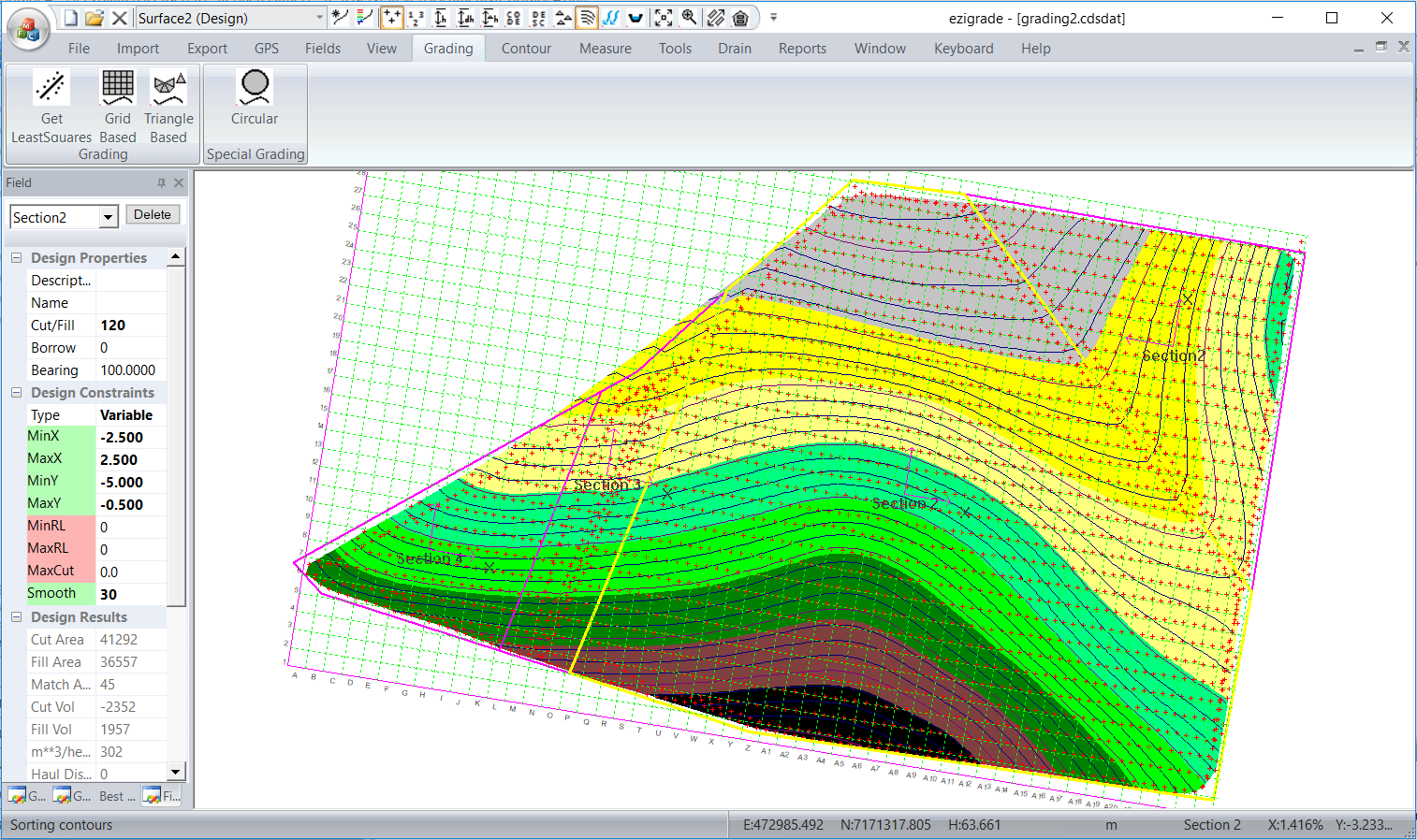TODO: Change the footer's content in Project Settings : HTML (CHM) Export : Setup HTML Template and Layout